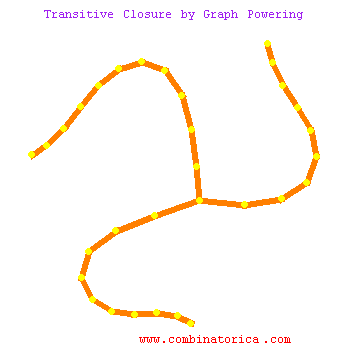## Transitive Closure by Graph Powering

The transitive closure T(G) of a given graph G connects vertices u and v iff there is a path in G from u to v. Thus the transitive closure of any connected graph is complete.

This animation finds the transitive closure of a graph by taking its adjacency matrix and raising it to the nth power, where n is the number of vertices in G. When we raise the graph to the kth power, we add exactly the edges which represent paths of length k in the original graph.

In this animation, red denotes most recently added edges, blue the edges added the previous iteration, and green the edges two iterations before, before the edges cool to black. The original graph edges are shown in orange. A computationally cheaper way to find transitive closure is to use Warshall's algorithm, but graph powering also allows us to count how many paths there are of different lengths.